Anzeige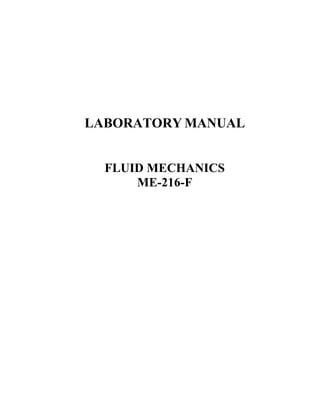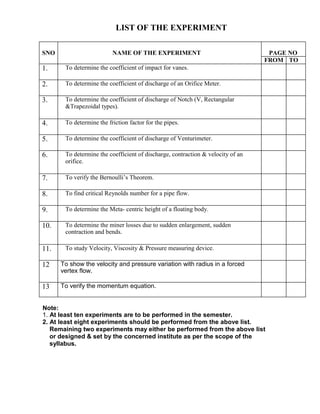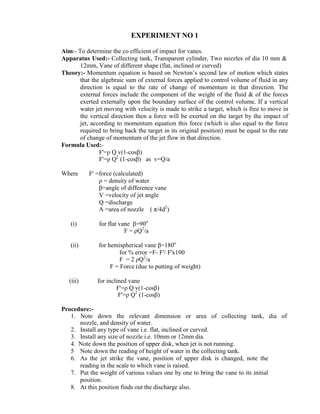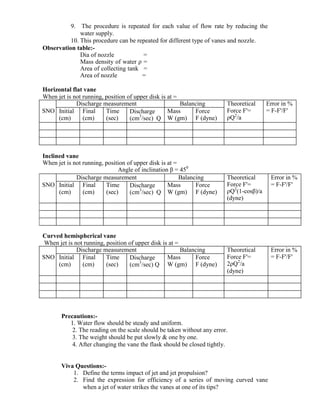Anzeige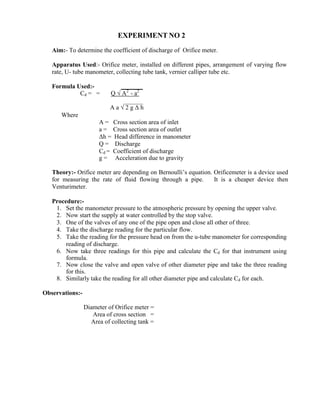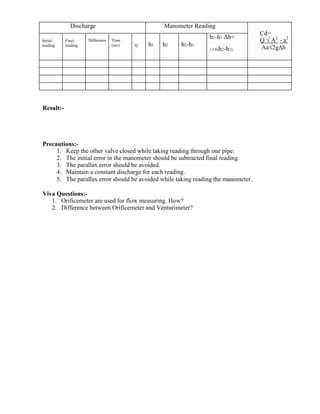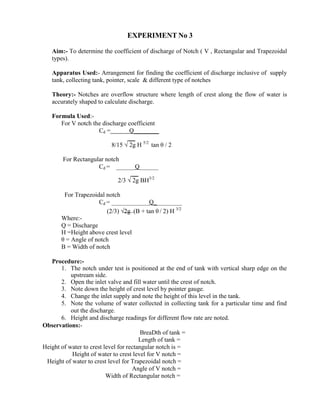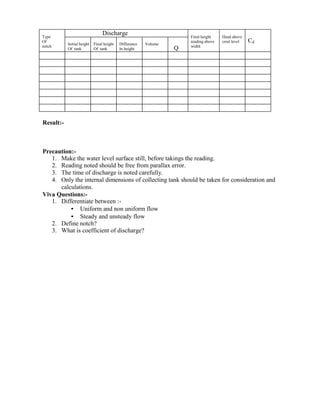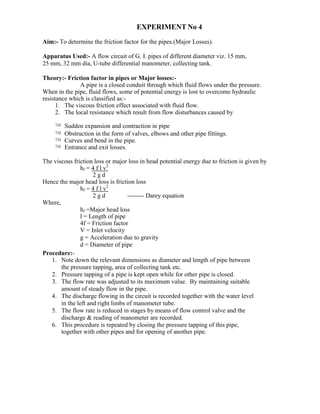Anzeige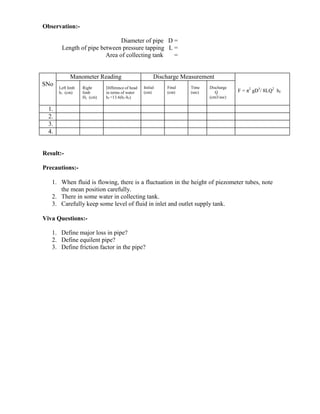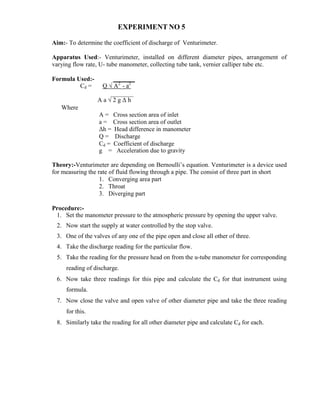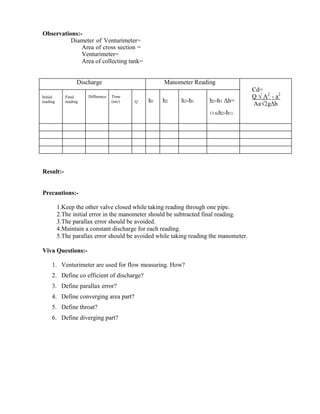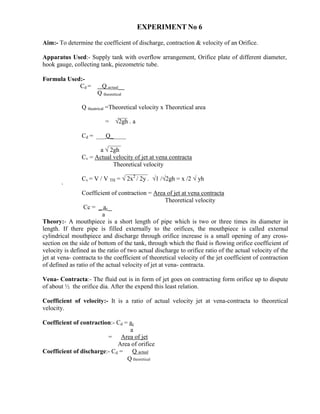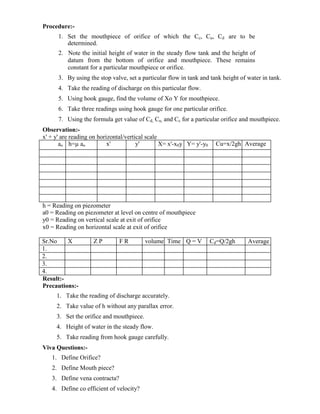Anzeige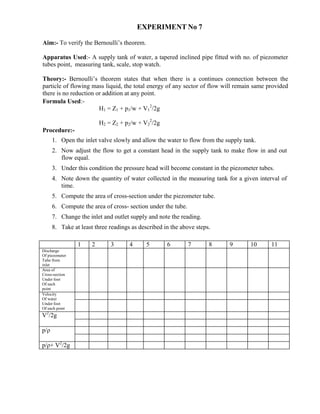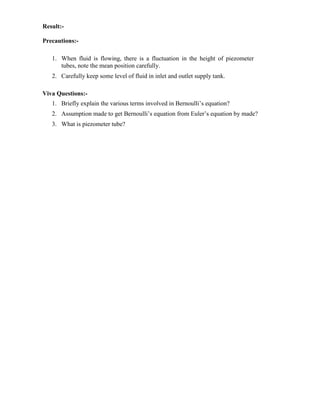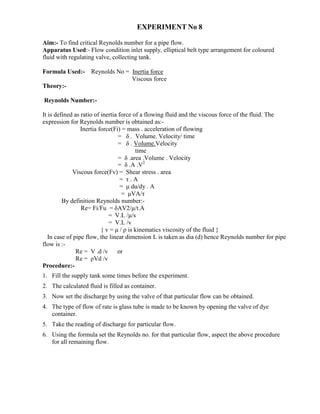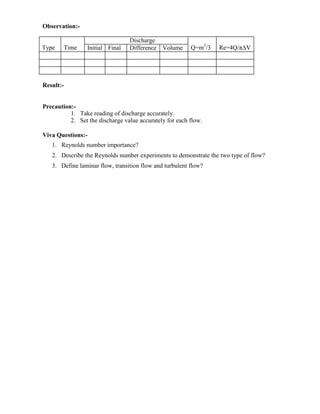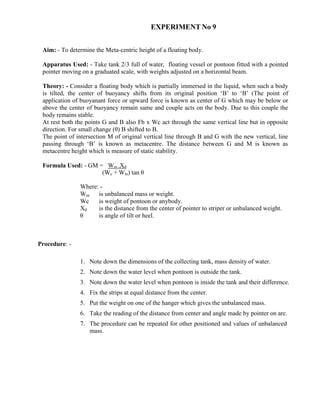Anzeige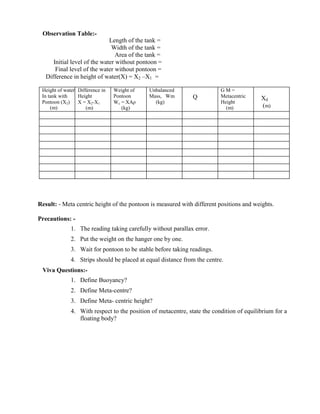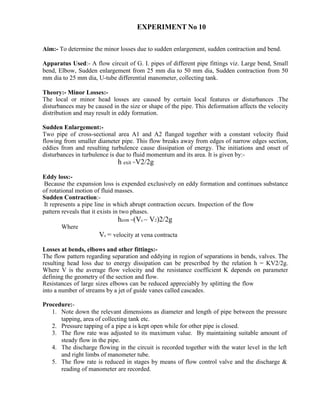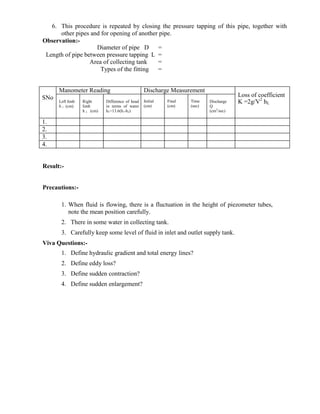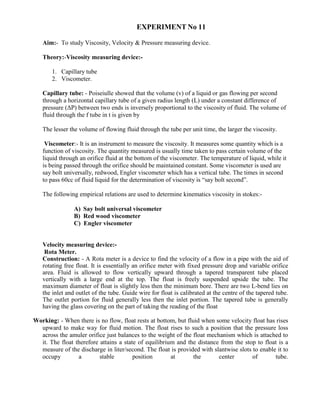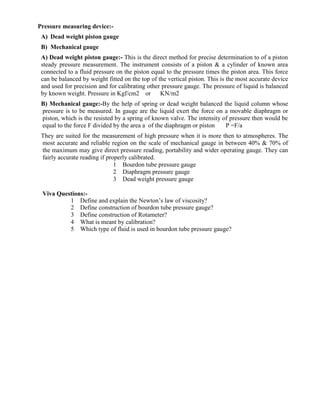Anzeige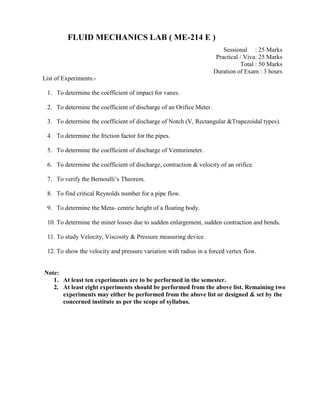Nächste SlideShare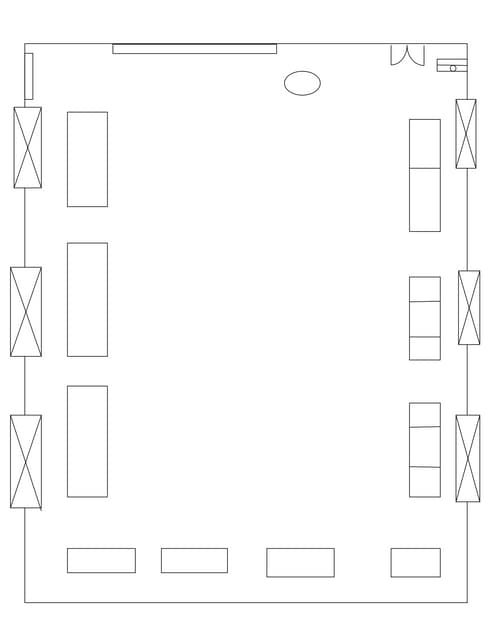Energy losses in Bends, loss coefficient related to velocity head.Pelton Whee...
1 von 25
Anzeige

### Fluid_Mechanics_Lab_IVSem (1).pdf

1. LABORATORY MANUAL FLUID MECHANICS ME-216-F
2. LIST OF THE EXPERIMENT SNO NAME OF THE EXPERIMENT PAGE NO FROM TO 1. To determine the coefficient of impact for vanes. 2. To determine the coefficient of discharge of an Orifice Meter. 3. To determine the coefficient of discharge of Notch (V, Rectangular &Trapezoidal types). 4. To determine the friction factor for the pipes. 5. To determine the coefficient of discharge of Venturimeter. 6. To determine the coefficient of discharge, contraction & velocity of an orifice. 7. To verify the Bernoulli’s Theorem. 8. To find critical Reynolds number for a pipe flow. 9. To determine the Meta- centric height of a floating body. 10. To determine the miner losses due to sudden enlargement, sudden contraction and bends. 11. To study Velocity, Viscosity & Pressure measuring device. 12 To show the velocity and pressure variation with radius in a forced vertex flow. 13 To verify the momentum equation. Note: 1. At least ten experiments are to be performed in the semester. 2. At least eight experiments should be performed from the above list. Remaining two experiments may either be performed from the above list or designed & set by the concerned institute as per the scope of the syllabus.
3. EXPERIMENT NO 1 Aim:- To determine the co efficient of impact for vanes. Apparatus Used:- Collecting tank, Transparent cylinder, Two nozzles of dia 10 mm & 12mm, Vane of different shape (flat, inclined or curved) Theory:- Momentum equation is based on Newton’s second law of motion which states that the algebraic sum of external forces applied to control volume of fluid in any direction is equal to the rate of change of momentum in that direction. The external forces include the component of the weight of the fluid & of the forces exerted externally upon the boundary surface of the control volume. If a vertical water jet moving with velocity is made to strike a target, which is free to move in the vertical direction then a force will be exerted on the target by the impact of jet, according to momentum equation this force (which is also equal to the force required to bring back the target in its original position) must be equal to the rate of change of momentum of the jet flow in that direction. Formula Used:- F'=ρ Q v(1-cosβ) F'=ρ Q2 (1-cosβ) as v=Q/a Where F' =force (calculated) ρ = density of water β=angle of difference vane V =velocity of jet angle Q =discharge A =area of nozzle ( π/4d2 ) (i) for flat vane β=90o F = ρQ2 /a (ii) for hemispherical vane β=180o for % error =F- F'/ F'x100 F = 2 ρQ2 /a F = Force (due to putting of weight) (iii) for inclined vane F'=ρ Q v(1-cosβ) F'=ρ Q2 (1-cosβ) Procedure:- 1. Note down the relevant dimension or area of collecting tank, dia of nozzle, and density of water. 2. Install any type of vane i.e. flat, inclined or curved. 3. Install any size of nozzle i.e. 10mm or 12mm dia. 4. Note down the position of upper disk, when jet is not running. 5 Note down the reading of height of water in the collecting tank. 6. As the jet strike the vane, position of upper disk is changed, note the reading in the scale to which vane is raised. 7. Put the weight of various values one by one to bring the vane to its initial position. 8. At this position finds out the discharge also.
4. SNO Discharge measurement Balancing Theoretical Force F'= ρQ2 (1-cosβ)/a (dyne) Error in % = F-F'/F' Initial (cm) Final (cm) Time (sec) Discharge (cm3 /sec) Q Mass W (gm) Force F (dyne) 9. The procedure is repeated for each value of flow rate by reducing the water supply. 10. This procedure can be repeated for different type of vanes and nozzle. Observation table:- Dia of nozzle = Mass density of water ρ = Area of collecting tank = Area of nozzle = Horizontal flat vane When jet is not running, position of upper disk is at = SNO Discharge measurement Balancing Theoretical Force F'= ρQ2 /a Error in % = F-F'/F' Initial (cm) Final (cm) Time (sec) Discharge (cm3 /sec) Q Mass W (gm) Force F (dyne) Inclined vane When jet is not running, position of upper disk is at = Angle of inclination β = 450 Curved hemispherical vane When jet is not running, position of upper disk is at = SNO Discharge measurement Balancing Theoretical Force F'= 2ρQ2 /a (dyne) Error in % = F-F'/F' Initial (cm) Final (cm) Time (sec) Discharge (cm3 /sec) Q Mass W (gm) Force F (dyne) Precautions:- 1. Water flow should be steady and uniform. 2. The reading on the scale should be taken without any error. 3. The weight should be put slowly & one by one. 4. After changing the vane the flask should be closed tightly. Viva Questions:- 1. Define the terms impact of jet and jet propulsion? 2. Find the expression for efficiency of a series of moving curved vane when a jet of water strikes the vanes at one of its tips?
5. EXPERIMENT NO 2 Aim:- To determine the coefficient of discharge of Orifice meter. Apparatus Used:- Orifice meter, installed on different pipes, arrangement of varying flow rate, U- tube manometer, collecting tube tank, vernier calliper tube etc. Formula Used:- Cd = = Q √ A2 - a2 A a √ 2 g Δ h Where A = Cross section area of inlet a = Cross section area of outlet Δh = Head difference in manometer Q = Discharge Cd = Coefficient of discharge g = Acceleration due to gravity Theory:- Orifice meter are depending on Bernoulli’s equation. Orificemeter is a device used for measuring the rate of fluid flowing through a pipe. It is a cheaper device then Venturimeter. Procedure:- 1. Set the manometer pressure to the atmospheric pressure by opening the upper valve. 2. Now start the supply at water controlled by the stop valve. 3. One of the valves of any one of the pipe open and close all other of three. 4. Take the discharge reading for the particular flow. 5. Take the reading for the pressure head on from the u-tube manometer for corresponding reading of discharge. 6. Now take three readings for this pipe and calculate the Cd for that instrument using formula. 7. Now close the valve and open valve of other diameter pipe and take the three reading for this. 8. Similarly take the reading for all other diameter pipe and calculate Cd for each. Observations:- Diameter of Orifice meter = Area of cross section = Area of collecting tank =
6. Discharge Manometer Reading Cd= Q √ A2 - a2 Aa√2g∆h Initial reading Final reading Difference Time (sec) Q h1 h2 h2-h1 h2-h1 Δh= 13.6(h2-h1) Result:- Precautions:- 1. Keep the other valve closed while taking reading through one pipe. 2. The initial error in the manometer should be subtracted final reading. 3. The parallax error should be avoided. 4. Maintain a constant discharge for each reading. 5. The parallax error should be avoided while taking reading the manometer. Viva Questions:- 1. Orificemeter are used for flow measuring. How? 2. Difference between Orificemeter and Venturimeter?
7. EXPERIMENT No 3 Aim:- To determine the coefficient of discharge of Notch ( V , Rectangular and Trapezoidal types). Apparatus Used:- Arrangement for finding the coefficient of discharge inclusive of supply tank, collecting tank, pointer, scale & different type of notches Theory:- Notches are overflow structure where length of crest along the flow of water is accurately shaped to calculate discharge. Formula Used:- For V notch the discharge coefficient Cd = Q 8/15 √ 2g H 5/2 tan θ / 2 For Rectangular notch Cd = Q 2/3 √ 2g BH3/2 For Trapezoidal notch Cd = Q_ Where:- Q = Discharge (2/3) √2g (B + tan θ / 2) H 3/2 H =Height above crest level θ = Angle of notch B = Width of notch Procedure:- 1. The notch under test is positioned at the end of tank with vertical sharp edge on the upstream side. 2. Open the inlet valve and fill water until the crest of notch. 3. Note down the height of crest level by pointer gauge. 4. Change the inlet supply and note the height of this level in the tank. 5. Note the volume of water collected in collecting tank for a particular time and find out the discharge. 6. Height and discharge readings for different flow rate are noted. Observations:- BreaDth of tank = Length of tank = Height of water to crest level for rectangular notch is = Height of water to crest level for V notch = Height of water to crest level for Trapezoidal notch = Angle of V notch = Width of Rectangular notch =
8. Type Of notch Discharge Final height reading above width Head above crest level Cd Initial height Of tank Final height Of tank Difference In height Volume Q Result:- Precaution:- 1. Make the water level surface still, before takings the reading. 2. Reading noted should be free from parallax error. 3. The time of discharge is noted carefully. 4. Only the internal dimensions of collecting tank should be taken for consideration and calculations. Viva Questions:- 1. Differentiate between :- • Uniform and non uniform flow • Steady and unsteady flow 2. Define notch? 3. What is coefficient of discharge?
9. EXPERIMENT No 4 Aim:- To determine the friction factor for the pipes.(Major Losses). Apparatus Used:- A flow circuit of G. I. pipes of different diameter viz. 15 mm, 25 mm, 32 mm dia, U-tube differential manometer, collecting tank. Theory:- Friction factor in pipes or Major losses:- A pipe is a closed conduit through which fluid flows under the pressure. When in the pipe, fluid flows, some of potential energy is lost to overcome hydraulic resistance which is classified as:- 1. The viscous friction effect associated with fluid flow. 2. The local resistance which result from flow disturbances caused by ™ Sudden expansion and contraction in pipe ™ Obstruction in the form of valves, elbows and other pipe fittings. ™ Curves and bend in the pipe. ™ Entrance and exit losses. The viscous friction loss or major loss in head potential energy due to friction is given by hf = 4 f l v2 2 g d Hence the major head loss is friction loss hf = 4 f l v2 2 g d -------- Darey equation Where, Procedure:- hf =Major head loss l = Length of pipe 4f = Friction factor V = Inlet velocity g = Acceleration due to gravity d = Diameter of pipe 1. Note down the relevant dimensions as diameter and length of pipe between the pressure tapping, area of collecting tank etc. 2. Pressure tapping of a pipe is kept open while for other pipe is closed. 3. The flow rate was adjusted to its maximum value. By maintaining suitable amount of steady flow in the pipe. 4. The discharge flowing in the circuit is recorded together with the water level in the left and right limbs of manometer tube. 5. The flow rate is reduced in stages by means of flow control valve and the discharge & reading of manometer are recorded. 6. This procedure is repeated by closing the pressure tapping of this pipe, together with other pipes and for opening of another pipe.
10. Observation:- Diameter of pipe D = Length of pipe between pressure tapping L = Area of collecting tank = SNo Manometer Reading Discharge Measurement F = π2 gD5 / 8LQ2 hf Left limb h1 (cm) Right limb H2 (cm) Difference of head in terms of water hf =13.6(h1-h2) Initial (cm) Final (cm) Time (sec) Discharge Q (cm3/sec) 1. 2. 3. 4. Result:- Precautions:- 1. When fluid is flowing, there is a fluctuation in the height of piezometer tubes, note the mean position carefully. 2. There in some water in collecting tank. 3. Carefully keep some level of fluid in inlet and outlet supply tank. Viva Questions:- 1. Define major loss in pipe? 2. Define equilent pipe? 3. Define friction factor in the pipe?
11. EXPERIMENT NO 5 Aim:- To determine the coefficient of discharge of Venturimeter. Apparatus Used:- Venturimeter, installed on different diameter pipes, arrangement of varying flow rate, U- tube manometer, collecting tube tank, vernier calliper tube etc. Formula Used:- Cd = Q √ A2 - a2 A a √ 2 g Δ h Where A = Cross section area of inlet a = Cross section area of outlet Δh = Head difference in manometer Q = Discharge Cd = Coefficient of discharge g = Acceleration due to gravity Theory:-Venturimeter are depending on Bernoulli’s equation. Venturimeter is a device used for measuring the rate of fluid flowing through a pipe. The consist of three part in short 1. Converging area part 2. Throat 3. Diverging part Procedure:- 1. Set the manometer pressure to the atmospheric pressure by opening the upper valve. 2. Now start the supply at water controlled by the stop valve. 3. One of the valves of any one of the pipe open and close all other of three. 4. Take the discharge reading for the particular flow. 5. Take the reading for the pressure head on from the u-tube manometer for corresponding reading of discharge. 6. Now take three readings for this pipe and calculate the Cd for that instrument using formula. 7. Now close the valve and open valve of other diameter pipe and take the three reading for this. 8. Similarly take the reading for all other diameter pipe and calculate Cd for each.
12. Observations:- Diameter of Venturimeter= Area of cross section = Venturimeter= Area of collecting tank= Discharge Manometer Reading Cd= Q √ A2 - a2 Aa√2g∆h Initial reading Final reading Difference Time (sec) Q h1 h2 h2-h1 h2-h1 Δh= 13.6(h2-h1) Result:- Precautions:- 1.Keep the other valve closed while taking reading through one pipe. 2.The initial error in the manometer should be subtracted final reading. 3.The parallax error should be avoided. 4.Maintain a constant discharge for each reading. 5.The parallax error should be avoided while taking reading the manometer. Viva Questions:- 1. Venturimeter are used for flow measuring. How? 2. Define co efficient of discharge? 3. Define parallax error? 4. Define converging area part? 5. Define throat? 6. Define diverging part?
13. EXPERIMENT No 6 Aim:- To determine the coefficient of discharge, contraction & velocity of an Orifice. Apparatus Used:- Supply tank with overflow arrangement, Orifice plate of different diameter, hook gauge, collecting tank, piezometric tube. Formula Used:- Cd = Q actual Q theoretical Q theatrical =Theoretical velocity x Theoretical area = √2gh . a Cd = Q_ a √ 2gh Cv = Actual velocity of jet at vena contracta Theoretical velocity Cv = V / V TH = √ 2x2 / 2y . √1 /√2gh = x /2 √ yh ` Coefficient of contraction = Area of jet at vena contracta Theoretical velocity Cc = _ ac a Theory:- A mouthpiece is a short length of pipe which is two or three times its diameter in length. If there pipe is filled externally to the orifices, the mouthpiece is called external cylindrical mouthpiece and discharge through orifice increase is a small opening of any cross- section on the side of bottom of the tank, through which the fluid is flowing orifice coefficient of velocity is defined as the ratio of two actual discharge to orifice ratio of the actual velocity of the jet at vena- contracta to the coefficient of theoretical velocity of the jet coefficient of contraction of defined as ratio of the actual velocity of jet at vena- contracta. Vena- Contracta:- The fluid out is in form of jet goes on contracting form orifice up to dispute of about ½ the orifice dia. After the expend this least relation. Coefficient of velocity:- It is a ratio of actual velocity jet at vena-contracta to theoretical velocity. Coefficient of contraction:- Cd = ac a = Area of jet Area of orifice Coefficient of discharge:- Cd = Q actual Q theoretical